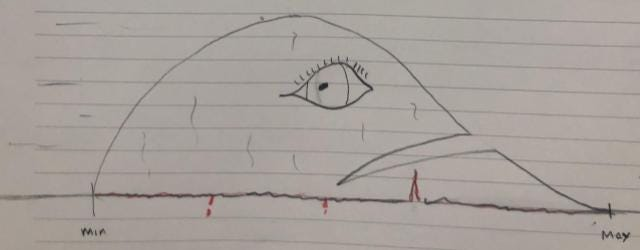The PERT distribution is a beta distribution, stretched out, ripped up and dumped down far from home. Poor thing. No actual beta distributions were harmed in preparing this article. All drawings by the author.

# ImPERTinence

For many, the PERT distribution is now the go-to distribution for encoding quantitative insights from subject matter experts.

But the PERT distribution is an ad hoc, belt-and-braces distribution determined far more by calculation convenience than any meaningful correspondence with the uncertainties we encounter. In this article, I will discuss the trouble that lurks both in the parameterization and the properties of the PERT distribution.

# What is the PERT distribution?

For a comprehensive introduction to the PERT distribution as well as an insightful discussion of its origins, see Stephen Grey’s excellent article here.

The PERT distribution describes the uncertainty on a variable that takes values between a prescribed minimum and maximum and that has its “most likely” value at a given mode.

(Aside: the probability of attaining exactly the “most likely” value is of course exactly zero, but it’s generally understood we mean the maximum of the density function.)

To make a PERT distribution, find a beta distribution with the right shape;

stretch it so that it has the right width;

then slice it off its axis and put it down so its back end sits at the required minimum.The PERT distribution is a stretched and translated beta distribution. Poor thing.

Joking (and bad drawing) aside, a PERT distribution is just a beta distribution that has been racked and relocated so that it sits between the required minimum and maximum values.

## Racked, relocated and restrained

The beta distribution is superlatively well suited to describe uncertainties in fractions, percentages and all things that fall naturally between 0 and 1. Comfortably nestled in its natural environment, it is completely described by two parameters, a mean and a variance say, though there are elegant alternatives.

The required scaling factor and translation distance required to manhandle the PERT distribution into its new location requires two more parameters, so the PERT distribution needs four parameters in all.

But if we want to fit a distribution to a minimum, maximum and mode, we only have three parameters, so we need some additional constraint that effectively expresses one of the four parameters in terms of the other three.

The standard constraint is to require that the mean is a specific weighted average of the minimum, maximum and mode.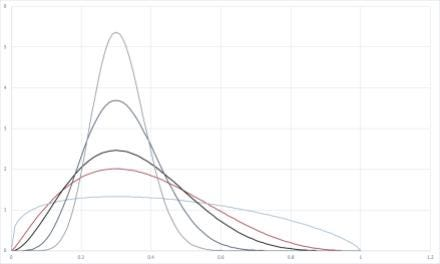A bunch of beta distributions with the same mode. All figures by the author. (See how well they look nestled comfortably between 0 and 1).

There are an infinite number of beta distributions with the minimum, mode and maximum in the right place. The constraint selects one for you (the red one in the figure).

## Standard deviation

The original perpetrator of the PERT distribution was looking to establish a standard deviation based on a minimum to maximum range. The ratio of the range to the standard deviation should, according to him, be around 6.

(Aside: This was based on the observation that 99.7% of all observations fall within three standard deviations either side of the mean of a normal distribution. Why 99.7% and not 99.99% (which would give a ratio of 8) or 99.9999% (10) isn’t clear. Nor why we should expect such characteristics to carry over from the symmetric normal distribution to these skewed beta distributions.)

The ratio condition can itself be made into a constraint (at the cost of dropping the constraint on the mean), but it is more usual to use the mean constraint and live with the standard deviation that gives you. This figure shows the ratio of the minimum-maximum range to the standard deviation for different values of the mode (expressed as the position of the mode as a proportion of the distance between the minimum and maximum). It doesn’t stray too far from 6.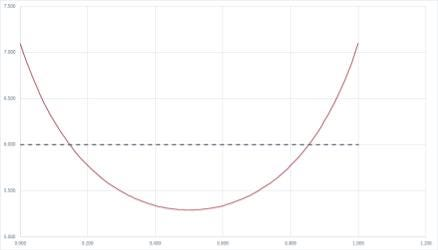The ratio of the minimum-maximum range to the standard deviation of a PERT distribution as a function of the position of the mode

# Problems with PERTs

I warned of trouble brewing. Over and above the cruel and unusual mistreatment of otherwise innocent beta distributions, there are four major issues using the PERT distribution.

1. Minimum and maximum are problematic parameters with which to parameterize any distribution.
2. The mode is a problematic parameter with which to parameterize any distribution.
3. PERT distributions scale awkwardly.
4. The variance is effectively too small for many practical applications because PERTs don’t have long tails, fat or otherwise.

# Parameterizing at the edge of distributions

I have written an entire article about the perils of parameterizing distributions with extreme tail values like the 1st or 99th percentile, and tail values don’t get more extreme than the maximum and minimum. There are two complementary challenges.

First, the shapes of distribution tails are nearly always governed by different considerations from those that determine the properties of the bulk of the distribution. Secondly, by their nature, distribution tails are under-sampled, so you need a lot of data or a lot of experience to establish them reliably. You can never know whether the absence of observations of lower or higher values than your current sense of the minimum and maximum is because they don’t happen or because they haven’t happened.

Avoid extreme percentiles, especially the absolute extremes of maximum and minimum.

The 10th and 90th percentiles are a good compromise between being far enough out to capture the sense of a reasonable range and close enough to the centre to still have something to do with the bulk of the distribution and to have a fighting chance of having data or experience enough to estimate them.

# Mushy modes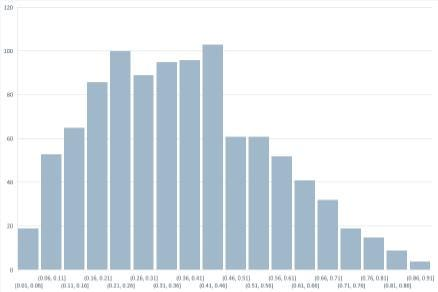Histogram of 1000 Monte Carlo iterations on a beta distribution with mode 0.3 and PERT mean. Figure by the author.

The figure here shows a 1000 iteration Monte Carlo simulation on the beta distribution with the red curve in the figure above. What’s the mode? Naively, based on these results, you might say around 0.45, which would be 150% in error. The mode of the distribution these are sampled from is 0.3.

Now 1000 iterations is a fairly modest Monte Carlo run, but if we imagine using subject matter experts with, generously, mental access to a couple of hundred exposures to the quantity they’re asked to assess, the situation is substantially worse.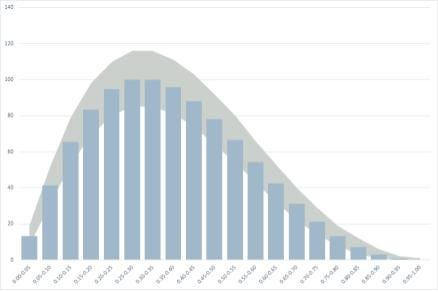Expected outcome rates for the same distribution with associated (90%) uncertainty range for a 1000 iteration Monte Carlo simulation

The problem is two-fold. First, the distribution is, by definition, flat at the mode, so small deviations in occurrence rates give potentially large errors in pinning down the value of the underlying variable at which those rates top out. The second problem is that the uncertainty around those occurrence rates is greatest at the mode. This you can see in the figure here, which shows the uncertainty range for the histogram bars in a 1000 iteration Monte Carlo simulation and illustrates the challenge of placing the mode, even on the basis of a lot of data points.

For the more representative couple of hundred points of reference, see the figure below.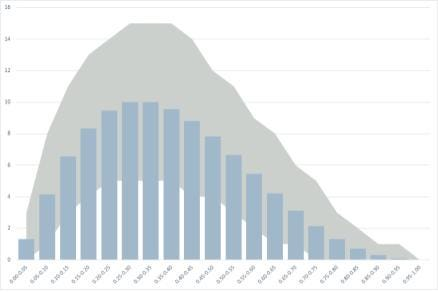Expected outcome rates and uncertainty range for a 100 iteration Monte Carlo distribution

If you have data, use the mean. If you are asking experts, use the median.

The mean is the statistic that converges the quickest with the number of data. The median is substantially more robust than the mode, and human beings are better at assessing medians than means because we struggle to account for the effect of long tails on the mean.

# Scaling

Take a PERT distribution with minimum a and maximum b. Suppose this represents a project phase duration. Without knowing its mode, I know that the standard deviation is roughly (b-a)/6 (variance (b-a)²/36).

If I have four consecutive project phases whose durations have the same distribution, then the minimum is now 4a and the maximum 4b, which would give a standard deviation of 4(b-a)/6.

But when we add random variables, we can add variances (the squares of the standard deviations), but not standard deviations. The variance of the 4 consecutive phases is then 4(b-a)²/36, so the standard deviation is actually 2(b-a)/6, half of what the PERT distribution implies.

Now, no one claims PERT distributions are additive, i.e. that the sum of two PERT distributions is a PERT distribution. The problem here is that the variance you get from an assessment is enormously dependent on whether you ask your expert to assess the individual project phase or the four identical phases together.

In truth, this is really a problem with parameterizing with maximum and minimum. It becomes much less of a problem if you use more reasonable outer bounds whose proximity to the actual extrema varies with the size of the range.

If you’re not interested in variance and you’re just using the distribution to establish means, then there are better ways of estimating means from three-point estimates. Swanson’s mean is not one of them, though; see my article Swanson’s Swansong.

# Not so variable variance and long tails

Left-skewed distributions with long tails, such as the log-normal distribution, can have very large variances owing to the low probability but high impact events in the long tail. Because the right tail of the PERT distribution is set to zero at the maximum value, its variance is necessarily constrained. Under all circumstances, we know the standard deviation won’t much exceed one-sixth of the minimum to maximum range.

In theory, we can always set the maximum-minimum range to be large enough to capture the full variance of any distribution (if it’s finite). The problem with doing so is that large variances require us to go out to very extreme percentiles. Apart from all the misery that brings in itself (see above), it tends also to distort the assessment of the mean.

In practice, we tend dramatically to underestimate how wide we need to make the PERT distribution in order to capture a large variance. As a result, we set the endpoints too narrowly and spectacularly fail to capture large variances in our assessments. This is a very common (and expensive) occurrence in oil and gas exploration.